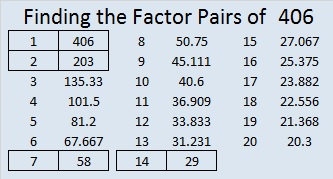# 406 and Level 4

Let’s apply the 7 divisibility trick to 406.

• Separate the last digit from the rest and multiply that last digit by two: 6 x 2 = 12.
• Now find the difference between the remaining number, 40 and 12: 40 – 12 = 28.
• Since 28 is a multiple of 7, we know that 406 is also divisible by 7.Print the puzzles or type the factors on this excel file: 12 Factors 2015-02-23

• 406 is a composite number.
• Prime factorization: 406 = 2 x 7 x 29
• The exponents in the prime factorization are 1, 1, and 1. Adding one to each and multiplying we get (1 + 1)(1 + 1)(1 + 1) = 2 x 2 x 2 = 8. Therefore 406 has exactly 8 factors.
• Factors of 406: 1, 2, 7, 14, 29, 58, 203, 406
• Factor pairs: 406 = 1 x 406, 2 x 203, 7 x 58, or 14 x 29
• 406 has no square factors that allow its square root to be simplified. √406 ≈ 20.1494## 4 thoughts on “406 and Level 4”

1.abyssbrain

For divisibility test for 7, we can also multiply the last digit by 5 (Since we are dealing with modulo)

6 x 5 = 30

40 + 30 = 70

70 is divisible by 7

•ivasallay

I didn’t know that! It’s good to know especially since most people would rather add than subtract.

•abyssbrain

There is a method for determining what number to multiply at the end of a number for divisibility tests of numbers like 7, 13 or 31. I may write a post about that later.

•ivasallay

That will indeed be an interesting post!

This site uses Akismet to reduce spam. Learn how your comment data is processed.